# Subtracting vectors

Subtracting - Reverse

Reverse the direction of the vector you want to subtract

Example 1

Subtract vector a  from vector b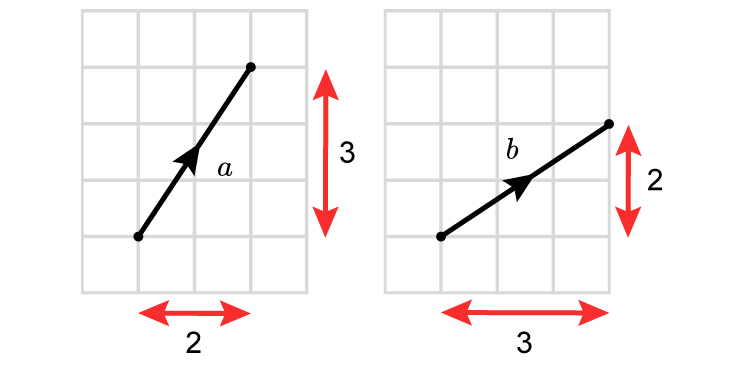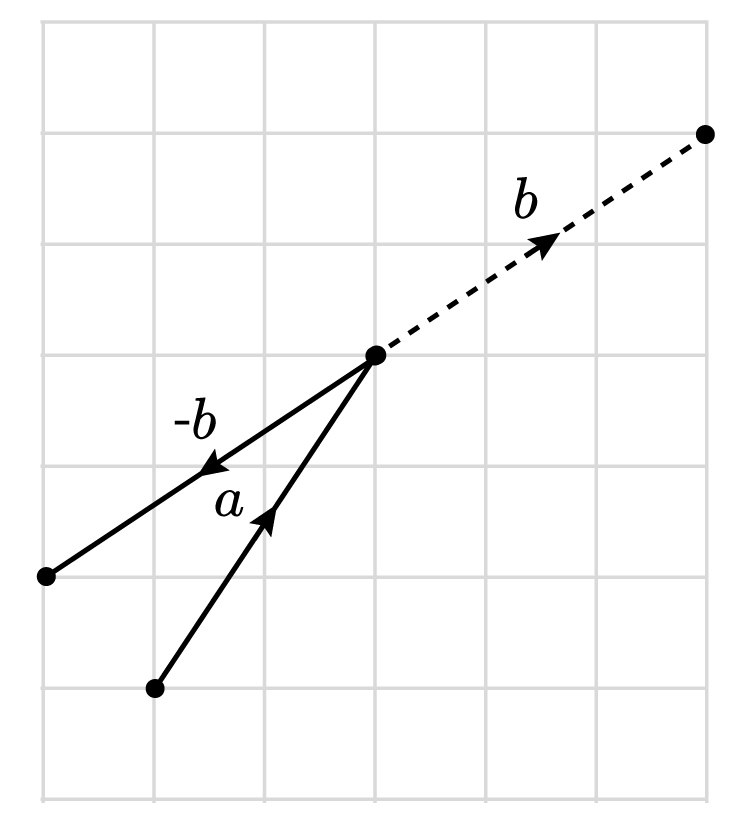Place the first vector a  and add on the reverse of vector b

The result is a new vector c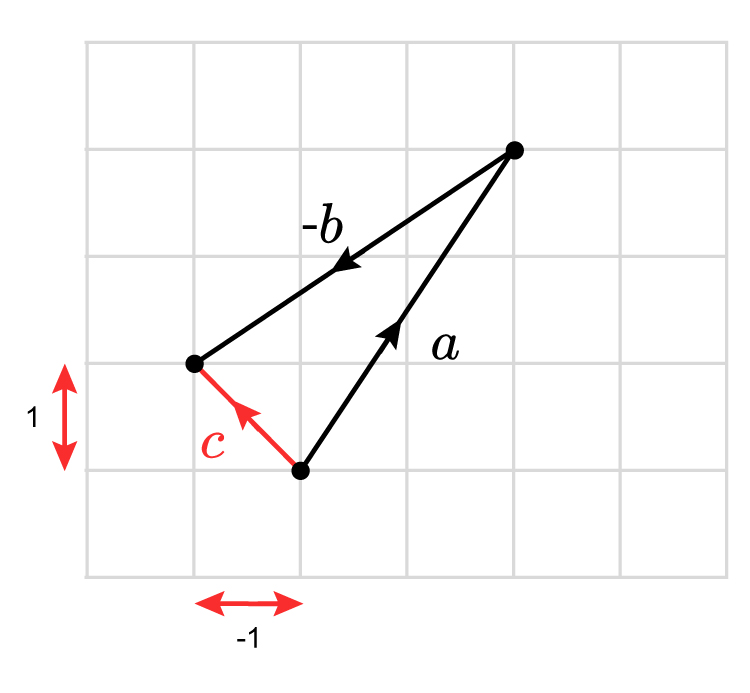NOTE:

It doesn't matter in which order you subtract vectors or add vectors. Therefore:

a-b=-b+a

But try -b+a and see for yourself.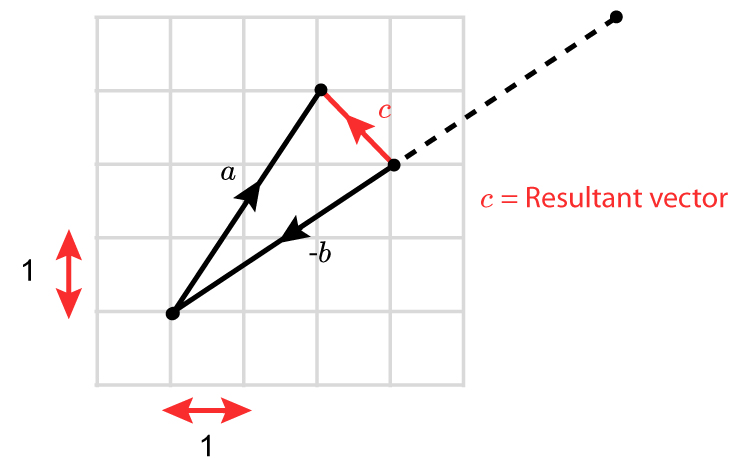a-b=c  and -b+a=c

Another way of showing a-b=-b+a  is as follows:

Example 2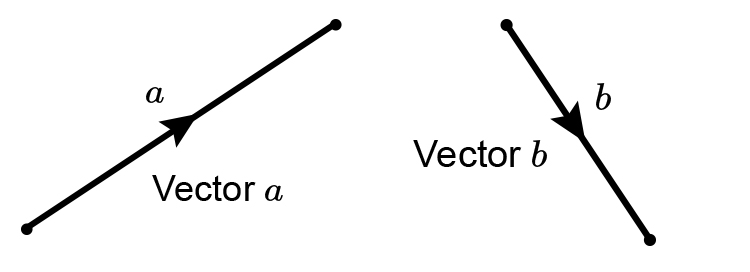a-b=-b+a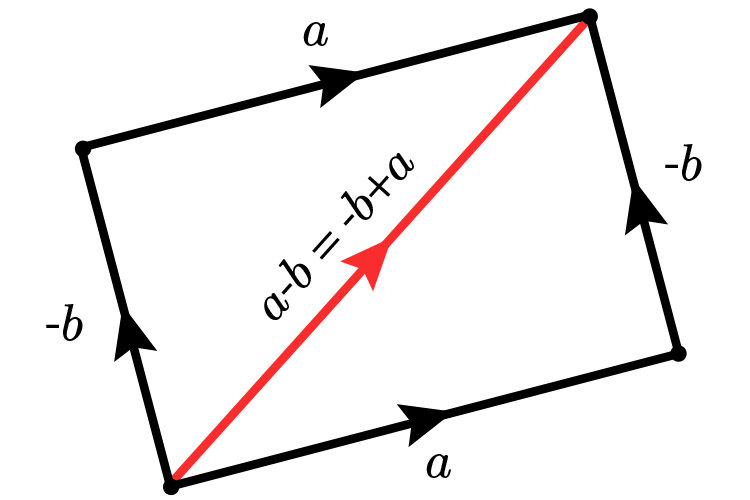Example 3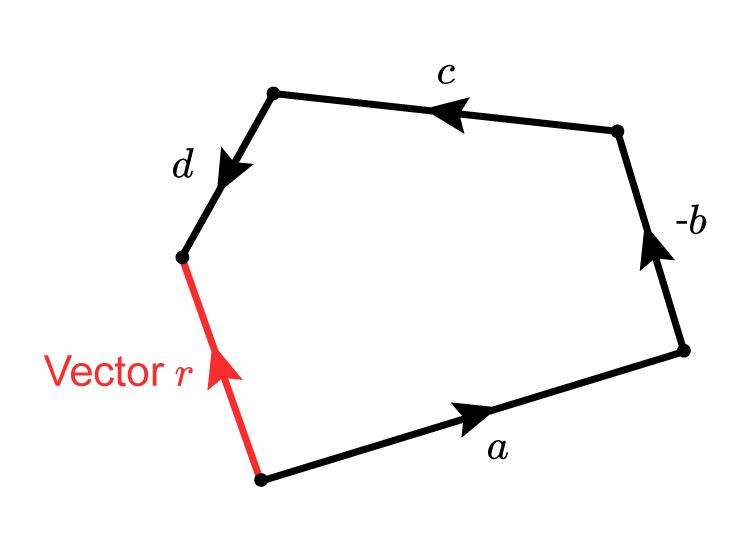Vector r=a-b+c+d

Also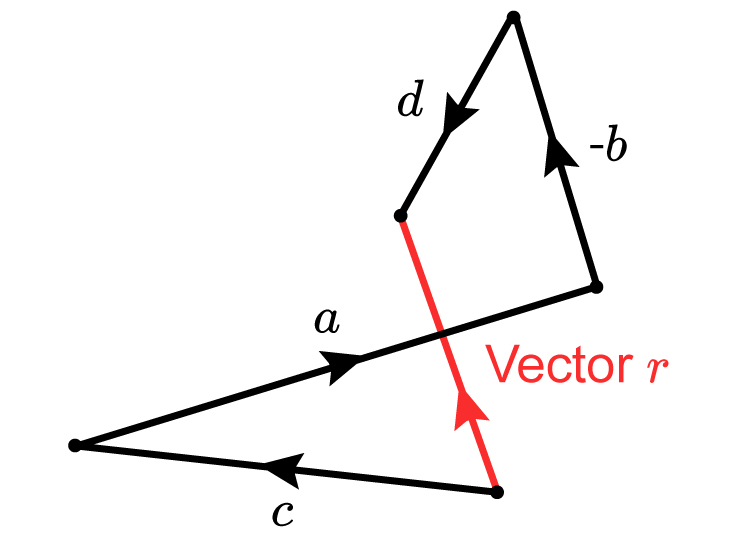Vector r=c+a-b+d

You can add vectors and reversed vectors in any order.